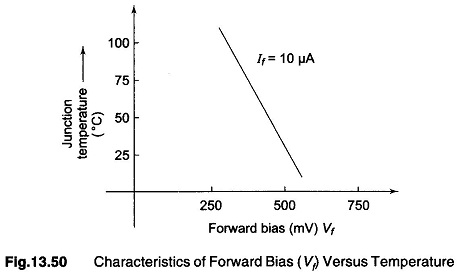## Semiconductor Temperature Sensing Diode:

Semiconductor Temperature Sensing Diode is a versatile device and finds use in many applications. Two of its parameter, that is forward voltage drop (Vf) and reverse saturation current (Is) are temperature sensitive. The sensitivity of Is with temperature is non-linear, but Vf has a linear temperature coefficient over a wide temperature range. Hence, Vf can serve as the basis for electronic thermometers.Figure 13.50 shows the characteristics of Vf versus temperature for a typical silicon pn junction diode. The linearity of this characteristics at high values of temperature is affected by the following factors.

1. Dependence of Vf on Is which is also temperature sensitive.
2. Presence of finite surface leakage component across the pn junction.
3. Finite resistance of the bulk semiconductor used for the temperature sensing diode.

The limitations introduced by point 1 can be overcomes by using a pair of well-matched junction transistors in a differential configuration to serve as the temperature sensors.

If the two transistors are operated at widely different emitter current values, the resulting VBE, Base emitter differential voltage can serve as an excellent index of temperature.

The association of differential mode voltage gain of this stage with VBE pro­vides means of adjusting the temperature coefficient to a desired value. This is the basis of IC type temperature transducer.

Scroll to Top